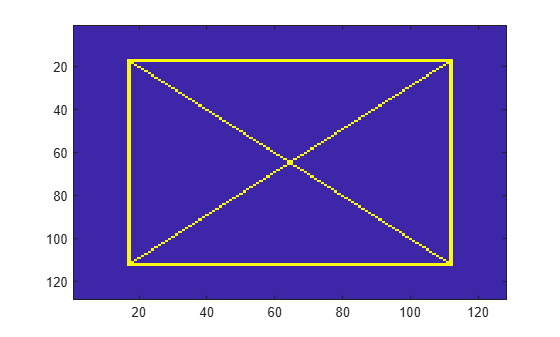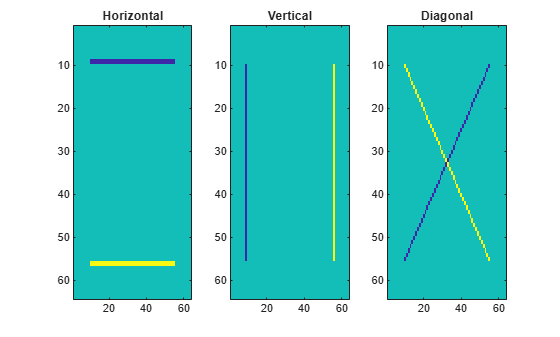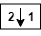# lwt2

2-D Lifting wavelet transform

## Syntax

``[ll,lh,hl,hh] = lwt2(x)``
``[___] = lwt2(x,Name=Value)``

## Description

example

````[ll,lh,hl,hh] = lwt2(x)` performs the 2-D lifting wavelet transform (LWT) of the real- or complex-valued matrix `x` using the `db1` wavelet. The function performs the decomposition first along the rows in `x` and then along the columns. The default decomposition level depends on the size of `x`. For more information, see Level. The function returns the approximation coefficients at the coarsest scale and the horizontal, vertical, and diagonal detail coefficients by level.If `x` is a single-precision input, the numeric type of the coefficients is single precision. Otherwise, the numeric type is double precision.```

example

````[___] = lwt2(x,Name=Value)` specifies options using one or more name-value arguments. For example, `lwt2(x,Wavelet="db2",Level=3)` performs 2-D LWT using the `db2` wavelet and a level 3 decomposition.```

## Examples

collapse all

Load and display the `xbox` image.

```load xbox imagesc(xbox)```Obtain the 2-D LWT of the image using default settings.

`[ll,lh,hl,hh] = lwt2(xbox);`

Display the first level detail coefficients.

```subplot(1,3,1) imagesc(lh{1}) title("Horizontal") subplot(1,3,2) imagesc(hl{1}) title("Vertical") subplot(1,3,3) imagesc(hh{1}) title("Diagonal")```Load an RGB image. An RGB image is also known as a truecolor image. The image is a 3-D array of type `uint8`.

```x = imread("ngc6543a.jpg"); image(x)```Create the lifting scheme associated with the `bior3.7` wavelet. Obtain the level 3 LWT of the image using the lifting scheme. Preserve the integer-valued data.

```lvl = 3; lScheme = liftingScheme("Wavelet","bior3.7"); [ll,lh,hl,hh] = lwt2(x,LiftingScheme=lScheme,Level=lvl,Int2Int=true);```

Confirm the approximation coefficients are all integer valued. Choose a level and confirm all the detail coefficients at that level are integer valued.

```approxDiffs = ll-floor(ll); max(abs(approxDiffs(:)))```
```ans = 0 ```
```lev = 2; horizDiffs = lh{lev}-floor(lh{lev}); vertDiffs = hl{lev}-floor(hl{lev}); diagDiffs = hh{lev}-floor(hh{lev}); [max(abs(horizDiffs(:))) max(abs(vertDiffs(:))) max(abs(diagDiffs(:)))]```
```ans = 1×3 0 0 0 ```

Reconstruct the image using the red and blue components of the coefficients. Display the reconstruction.

```llx = ll; llx(:,:,2) = 0; for k=1:lvl lhx{k} = lh{k}; hlx{k} = hl{k}; hhx{k} = hh{k}; lhx{k}(:,:,2) = 0; hlx{k}(:,:,2) = 0; hhx{k}(:,:,2) = 0; end xrec = ilwt2(llx,lhx,hlx,hhx,LiftingScheme=lScheme,Int2Int=true); imagesc(uint8(xrec)) title("Reconstruction")```Confirm the reconstruction is integer valued.

```recDiffs = xrec-floor(xrec); max(abs(recDiffs(:)))```
```ans = 0 ```

## Input Arguments

collapse all

Input data, specified as a real- or complex-valued 2-D, 3-D, or 4-D matrix. The input `x` must have at least two samples in the row and column dimensions.

• If `size(x,1)` is odd, the function extends `x` by duplicating the last row.

• If `size(x,2)` is odd, the function extends the last column of `x` by duplicating the last column.

Data Types: `single` | `double`
Complex Number Support: Yes

### Name-Value Arguments

Specify optional pairs of arguments as `Name1=Value1,...,NameN=ValueN`, where `Name` is the argument name and `Value` is the corresponding value. Name-value arguments must appear after other arguments, but the order of the pairs does not matter.

Example: ```[ll,lh,hl,hh] = lwt2(x,LiftingScheme=lscheme,Level=2)```

Orthogonal or biorthogonal wavelet to use in the 2-D LWT, specified as a character vector or string scalar. See the Wavelet property of `liftingScheme` for the list of supported wavelets.

You cannot specify `Wavelet` and `LiftingScheme` at the same time.

Example: `[ll,~,~,hh] = lwt2(x,Wavelet="bior3.5")` uses the `bior3.5` biorthogonal wavelet.

Data Types: `char` | `string`

Lifting scheme to use in the 2-D LWT, specified as a `liftingScheme` object.

You cannot specify `LiftingScheme` and `Wavelet` at the same time.

Example: `[~,lh,hl,~] = lwt2(x,LiftingScheme=lScheme)` uses the `lScheme` lifting scheme.

Decomposition level of the 2-D LWT, specified as a positive integer less than or equal to `floor(log2(N))`, where ```N = min(size(x,[1 2])/2)```.

The default decomposition level depends on the number of rows and columns in `x`.

• If the number of both the rows and the columns is a power of two, the function performs 2-D LWT down to level `log2(min(size(x,[1 2])))`.

• If the number of both the rows and the columns is even but at least one is not a power of two, the function performs 2-D LWT down to `floor(log2(N))`, where ```N = min(size(x,[1 2])/2)```.

Example: `[ll,~,hl,~] = lwt2(x,Level=4)` specifies a level 4 wavelet decomposition.

Data Types: `double`

Extension mode to use in the LWT, specified as one of these:

• `"periodic"` — Periodized extension

• `"zeropad"` — Zero extension

• `"symmetric"` — Symmetric extension

This argument specifies how `lwt2` extends the input at the boundaries.

Example: `[~,lh,~,hh] = lwt2(x,Extension="symmetric")` specifies the symmetric extension mode.

Data Types: `char` | `string`

Handling integer-valued data, specified as one of these:

• `1` (`true`) — Preserve integer-valued data

• `0` (`false`) — Do not preserve integer-valued data

Specify `Int2Int` only if all elements of the input are integers.

Example: `[~,lh,hl,hh] = lwt2(x,Int2Int=true)` preserves integer-valued data.

## Output Arguments

collapse all

Approximation coefficients at the coarsest scale, returned as a scalar, vector, or matrix.

Data Types: `single` | `double`

Horizontal detail coefficients by level, returned as a LEV-by-1 cell array, where LEV is the level of the decomposition. The elements of `lh` are in order of decreasing resolution.

Data Types: `single` | `double`

Vertical detail coefficients by level, returned as a LEV-by-1 cell array, where LEV is the level of the decomposition. The elements of `hl` are in order of decreasing resolution.

Data Types: `single` | `double`

Diagonal detail coefficients by level, returned as a LEV-by-1 cell array, where LEV is the level of the decomposition. The elements of `hh` are in order of decreasing resolution.

Data Types: `single` | `double`

## Algorithms

At each stage of a 2-D wavelet decomposition, the approximation coefficients at level j are decomposed into four components: the approximation at level j+1 and the details in three orientations (horizontal, vertical, and diagonal). Each component is the result of convolving the rows and columns of the level j approximation with the appropriate combination of lowpass and highpass filters, LoD and HiD, respectively, followed by downsampling:

• Approximation — Convolve the rows and columns with a lowpass filter (`ll`)

• Horizontal — Convolve the rows with a lowpass filter, and convolve the columns with a highpass filter (`lh`)

• Vertical — Convolve the rows with a highpass filter, and convolve the columns with a lowpass filter (`hl`)

• Diagonal — Convolve the rows and columns with a highpass filter (`hh`)

The following chart describes the basic decomposition steps.where

•— Downsample columns: keep the even-indexed columns

•— Downsample rows: keep the even-indexed rows

•— Convolve the rows of the entry with filter X

•— Convolve the columns of the entry with filter X

The decomposition is initialized by setting the approximation coefficients equal to the image s: cA0 = s.

 Daubechies, Ingrid. Ten Lectures on Wavelets. CBMS-NSF Regional Conference Series in Applied Mathematics 61. Philadelphia, Pa: Society for Industrial and Applied Mathematics, 1992.

 Mallat, S.G. “A Theory for Multiresolution Signal Decomposition: The Wavelet Representation.” IEEE Transactions on Pattern Analysis and Machine Intelligence 11, no. 7 (July 1989): 674–93. https://doi.org/10.1109/34.192463.

 Strang, Gilbert, and Truong Nguyen. Wavelets and Filter Banks. Rev. ed. Wellesley, Mass: Wellesley-Cambridge Press, 1997.

 Sweldens, Wim. “The Lifting Scheme: A Construction of Second Generation Wavelets.” SIAM Journal on Mathematical Analysis 29, no. 2 (March 1998): 511–46. https://doi.org/10.1137/S0036141095289051.